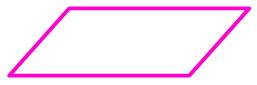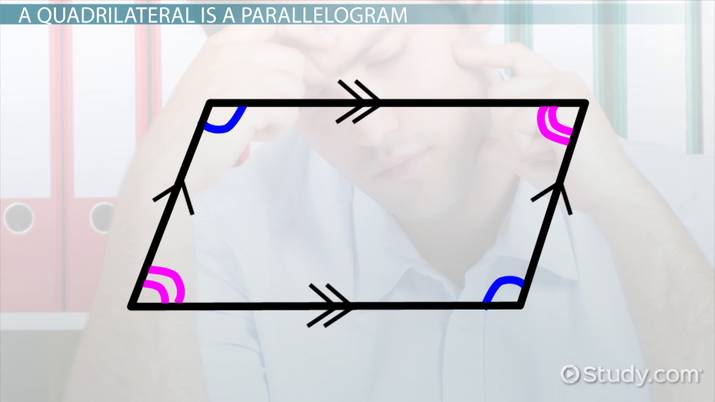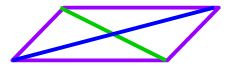# Proving That a Quadrilateral is a Parallelogram

Lesson Transcript
Instructor
Yuanxin (Amy) Yang Alcocer

Amy has a master's degree in secondary education and has been teaching math for over 9 years. Amy has worked with students at all levels from those with special needs to those that are gifted.

Expert Contributor
Laura Pennington

Laura received her Master's degree in Pure Mathematics from Michigan State University, and her Bachelor's degree in Mathematics from Grand Valley State University. She has 20 years of experience teaching collegiate mathematics at various institutions.

Learn how to prove when a quadrilateral, a shape with four sides, is a parallelogram, a polygon made of two sets of parallel lines. Discover the five methods using parallel lines, congruent lines, congruent angles, and diagonal measurements. Updated: 12/13/2021

## A Quadrilateral and a Parallelogram

When it comes to math, you have to be able to prove that what you're doing is correct. When it comes to geometry, it's the same. In geometry, you'll often be asked to prove that a certain shape is, indeed, that certain shape. For example, you might be shown a quadrilateral and be asked to prove that it is a parallelogram. Remember that a quadrilateral is a four-sided flat shape. A parallelogram is a quadrilateral with two pairs of opposite, parallel sides.

Looking at this shape, you might think that it is a parallelogram, but unless the problem specifically tells you and/or you can prove that it is, you can't say for sure that it's a parallelogram.This is where mathematical proofs are very important. You can only say for sure that this is a parallelogram with a mathematical proof. Most times, when you're asked to prove that a certain quadrilateral is a parallelogram, you'll be given information about just a few sides. It's then your job to prove that these sides have the right properties of a parallelogram. We'll be taking a closer look at this in a little bit.An error occurred trying to load this video.

Try refreshing the page, or contact customer support.

### You're on a roll. Keep up the good work!

Replay
Your next lesson will play in 10 seconds
• 0:00 A Quadrilateral and a…
• 1:08 A Quadrilateral Is a…
• 3:37 Example
• 6:15 Lesson Summary
Save Save

Want to watch this again later?

Timeline
Autoplay
Autoplay
Speed Speed

## A Quadrilateral Is a Parallelogram

But first, let's go over five ways you can use to prove that a quadrilateral is a parallelogram. Depending on what information you have to work with, you'll be using one of these five ways.

#### 1. Prove that both pairs of opposite sides are parallel.

This one is simply the reverse of the definition of a parallelogram. If you can prove that the quadrilateral fits the definition of a parallelogram, then it is a parallelogram.

#### 2. Prove that both pairs of opposite sides are congruent.

If both pairs of opposite sides of a quadrilateral are congruent, then you'll always have two opposite pairs of parallel sides. Congruent means that they measure the same. Think about it: two congruent sides separating the other pair of opposite sides must always keep those opposite lines the same distance apart. This means, then, that the opposite sides are also parallel.

#### 3. Prove that one pair of opposite sides is both congruent and parallel.

This one is kind of similar to the method before. It just goes about proving the case in another way. You can actually try this out with four toothpicks. Try it by placing two of the toothpicks opposite and parallel to each other. Now connect these two toothpicks at both ends with the other two toothpicks. You'll notice that no matter how you place your first pair of toothpicks, your second pair of toothpicks will always be parallel.

#### 4. Prove that the diagonals bisect each other (that they can divide each other into two equal parts where they cross).

This one is a bit harder to visualize. But you can play around with it by taking two different-sized sticks and crossing them in the middle of both sticks. These two sticks are the diagonals inside your parallelogram. You'll see that no matter how you cross your sticks, as long as they cross in the middle, you'll always get a parallelogram. Your two sticks are the blue and green lines. You can see that these are the diagonals inside the parallelogram.To unlock this lesson you must be a Study.com Member.

## Proving That a Quadrilateral is a Parallelogram

### Reminder

There are five ways to prove that a quadrilateral is a parallelogram:

• Prove that both pairs of opposite sides are congruent.
• Prove that both pairs of opposite sides are parallel.
• Prove that one pair of opposite sides is both congruent and parallel.
• Prove that the diagonals of the quadrilateral bisect each other.
• Prove that both pairs of opposite angles are congruent.

Once we have proven that one of these is true about a quadrilateral, we know that it is a parallelogram, so it satisfies all five of these properties of a parallelogram.

### Problems

1. A builder is building a modern TV stand. He starts with two beams that form an X-shape, such that they intersect at each other's midpoint. If he connects the endpoints of the beams with four straight wooden sides to create the TV stand, what shape will the TV stand be? If one of the wooden sides has a length of 2 feet, and another wooden side has a length of 3 feet, what are the lengths of the remaining wooden sides?
2. A race director has put together a marathon that runs on four straight roads. A marathon is 26.2 miles total, the four roads make up a quadrilateral, and the pairs of opposite angles created by those four roads have the same measure. What does this tell us about the shape of the course? If one of the roads is 4 miles, what are the lengths of the other roads?

### Solutions

1. Since the two beams form an X-shape, such that they intersect at each other's midpoint, we have that the two beams bisect one another, so if we connect the endpoints of these two beams with four straight wooden sides, it will create a quadrilateral with diagonals that bisect one another. Therefore, the wooden sides will be a parallelogram. Since parallelograms have opposite sides that are congruent, it must be the case that the side of length 2 feet has an opposite side of length 2 feet, and the side that has a length of 3 feet must have an opposite side with a length of 3 feet. Therefore, the lengths of the remaining wooden sides are 2 feet and 3 feet.
2. Since the four roads create a quadrilateral in which the opposite angles have the same measure (or are congruent), we have that the roads create a parallelogram. We know that a parallelogram has congruent opposite sides, and we know that one of the roads has a length of 4 miles. Thus, the road opposite this road also has a length of 4 miles. This makes up 8 miles total. There are 26.2 miles total in a marathon, so the remaining two roads must make up 26.2 - 8 = 18.2 miles of the race. Furthermore, the remaining two roads are opposite one another, so they have the same length. Therefore, the remaining two roads each have a length of one-half of 18.2, which is 9.1 miles. This gives that the four roads on the course have lengths of 4 miles, 4 miles, 9.1 miles, and 9.1 miles.

### Register to view this lesson

Are you a student or a teacher?

Back

### Resources created by teachers for teachers

Over 30,000 video lessons & teaching resources‐all in one place.Video lessonsQuizzes & WorksheetsClassroom IntegrationLesson Plans

I would definitely recommend Study.com to my colleagues. It’s like a teacher waved a magic wand and did the work for me. I feel like it’s a lifeline.

Jennifer B.
TeacherCreate an account to start this course today
Used by over 30 million students worldwide